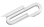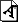Spring 2020

# Fri April 10

Continue our discussion of momentum.

resources :

Some classic problems. (Some of these are tricky.)

## ballistic pendulum

How can you measure the speed of a bullet?

One good way is to shoot the bullet into a block of something that that will stop it, like a block of wood, which is suspended so that it can swing as a pendulum. Then you measure how far the pendulum swings.

Make sure you understand what is conversed and what is not, at the various "interesting" times.

## block stacking problem

Place N identical rigid rectangular blocks in a stable stack on a table edge in such a way as to maximize the overhang.

discussion & answer : wikipedia: block-stacking problem

## Galilean cannon

aka "stacked ball drop"

Here's a youtube video of the experiment.

Your mission: analyze the collisions between two stacked balls as they drop and bounce. Let the bottom mass be 10 and the top be 1. Do the collisions in succession: first the bottom ball with the ground, then after that the top ball with the bottom. Assume elastic collisions for simplicity.

## 1D billiards

Consider three balls moving along a line in one dimension. The collisions between them are inelastic with some fraction of velocity lost (see coifficient of restitution).

What is the maximum number of collisions possible given any set of initial positions and velocities?

(This one I remember being posed at a physics conference I was at, and I obsessed over it afterwards ... there's a very cool answer and solution approach.)

## textbook problems we may do in class

• 11.38 - tennis racket hits a ball
• 11.48 - glancing billiard ball collision. (For extra fun: what is this in the center of mass coord system?)
• 11.52 - rocket explodes into two pieces

## in class

I've attached a pdf of the blackboard scrawl I did during class.

However, the K&K problem is wrong on that attached pdf. We looked at a correct worked solution and saw that I set (M + dm)(V+dv) to (M)(V+dv) incorrectly, which dropped a (dmV) term which was important, leading to a dm(u-V) term after doing momentum conversation.

https://cs.marlboro.college /cours /spring2020 /mechanics /notes /apr10
## attachmentslast modified sizeblackboard_physics_april10.pdf Sun Oct 17 2021 12:33 pm 938K